# NCERT Solutions for Class 8 Maths Chapter 8 Exercise 8.2 – Comparing Quantities

NCERT Solutions for Class 8 Maths Chapter 8 Exercise 8.2 – Comparing Quantities, has been designed by the NCERT to test the knowledge of the student on the following topics:-

• Finding the Increase or Decrease Per cent
• Finding Discounts
Estimation in percentages
• Prices Related to Buying and Selling (Profit and Loss)
Finding cost price/selling price, profit %/loss%
• Sales Tax/Value Added Tax/Goods and Services Tax

### NCERT Solutions for Class 8 Maths Chapter 8 Exercise 8.2 – Comparing Quantities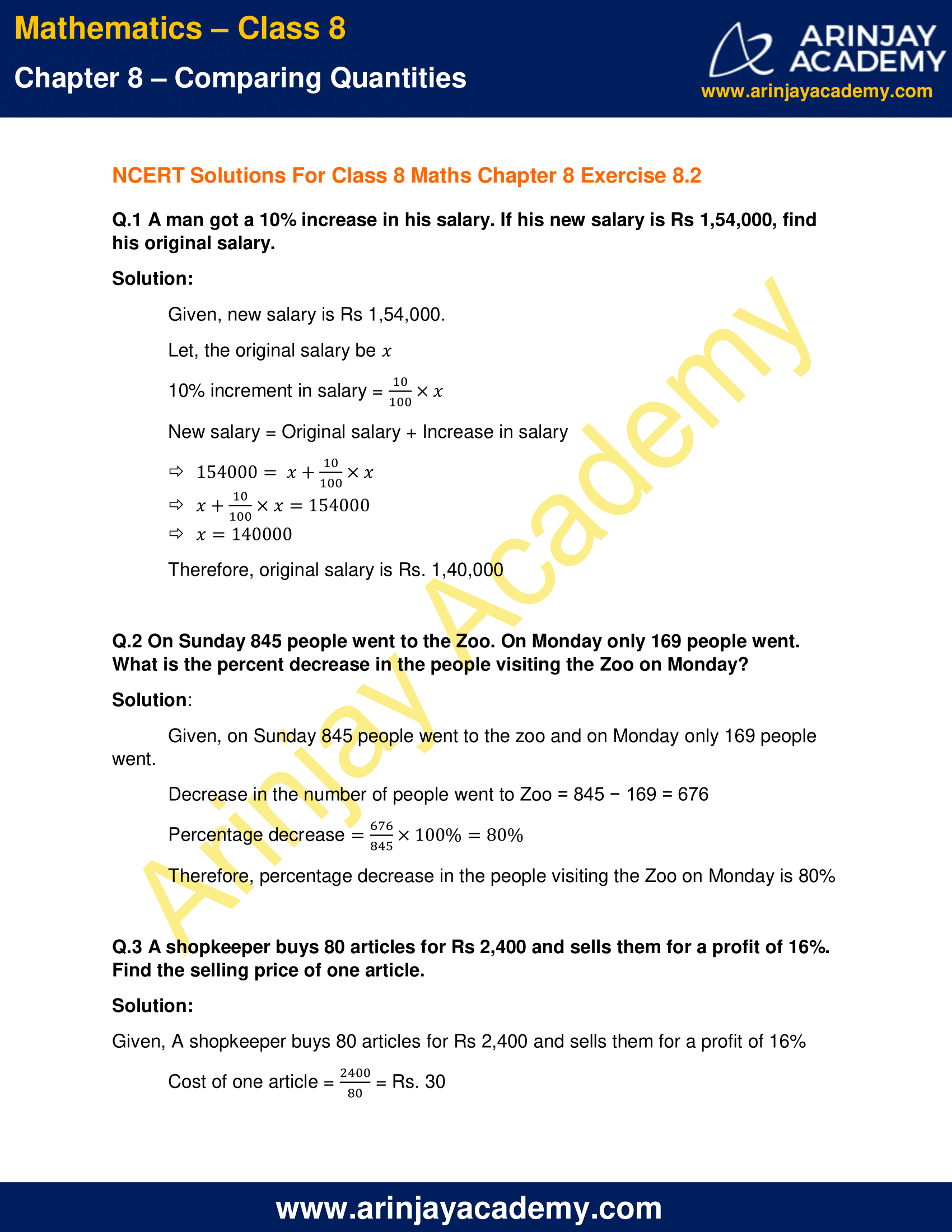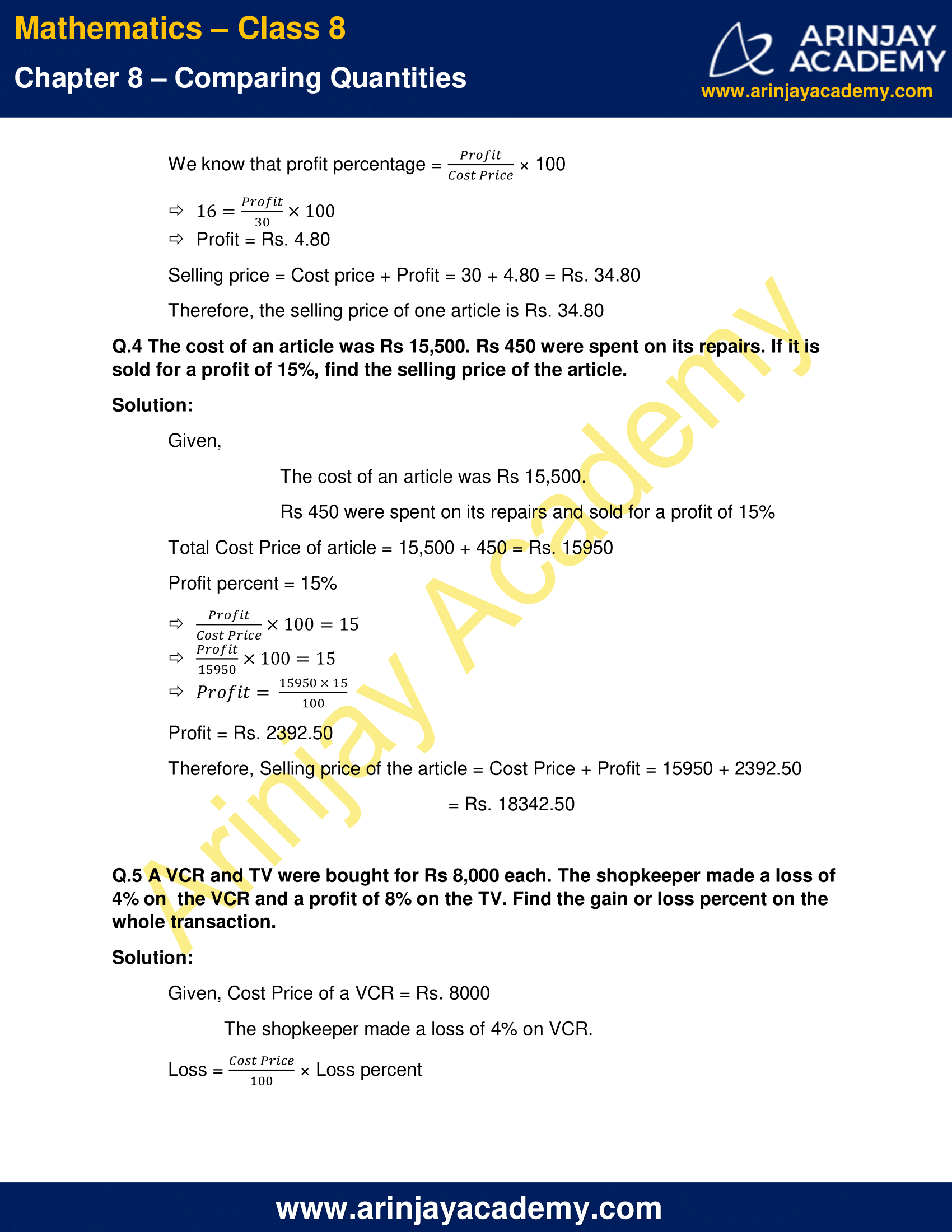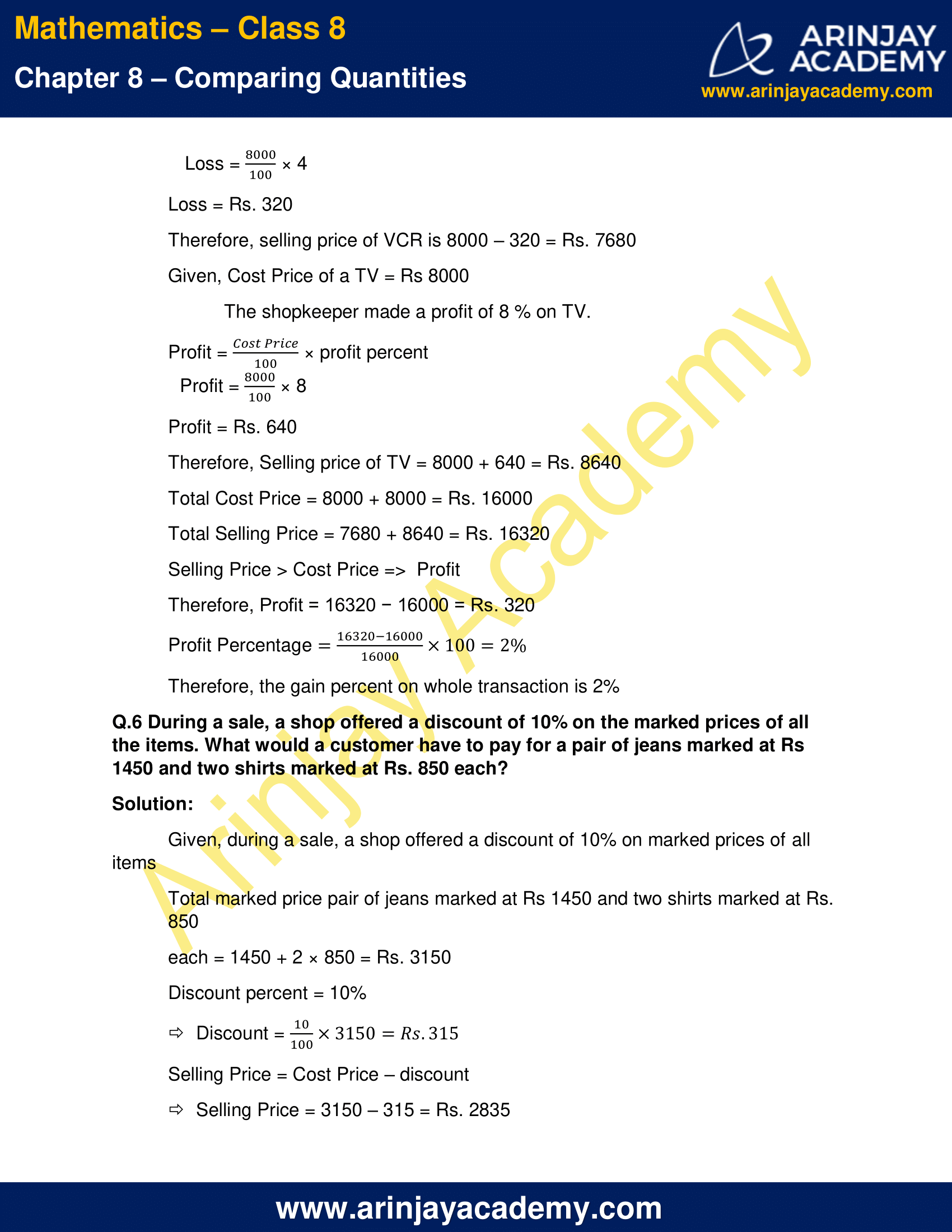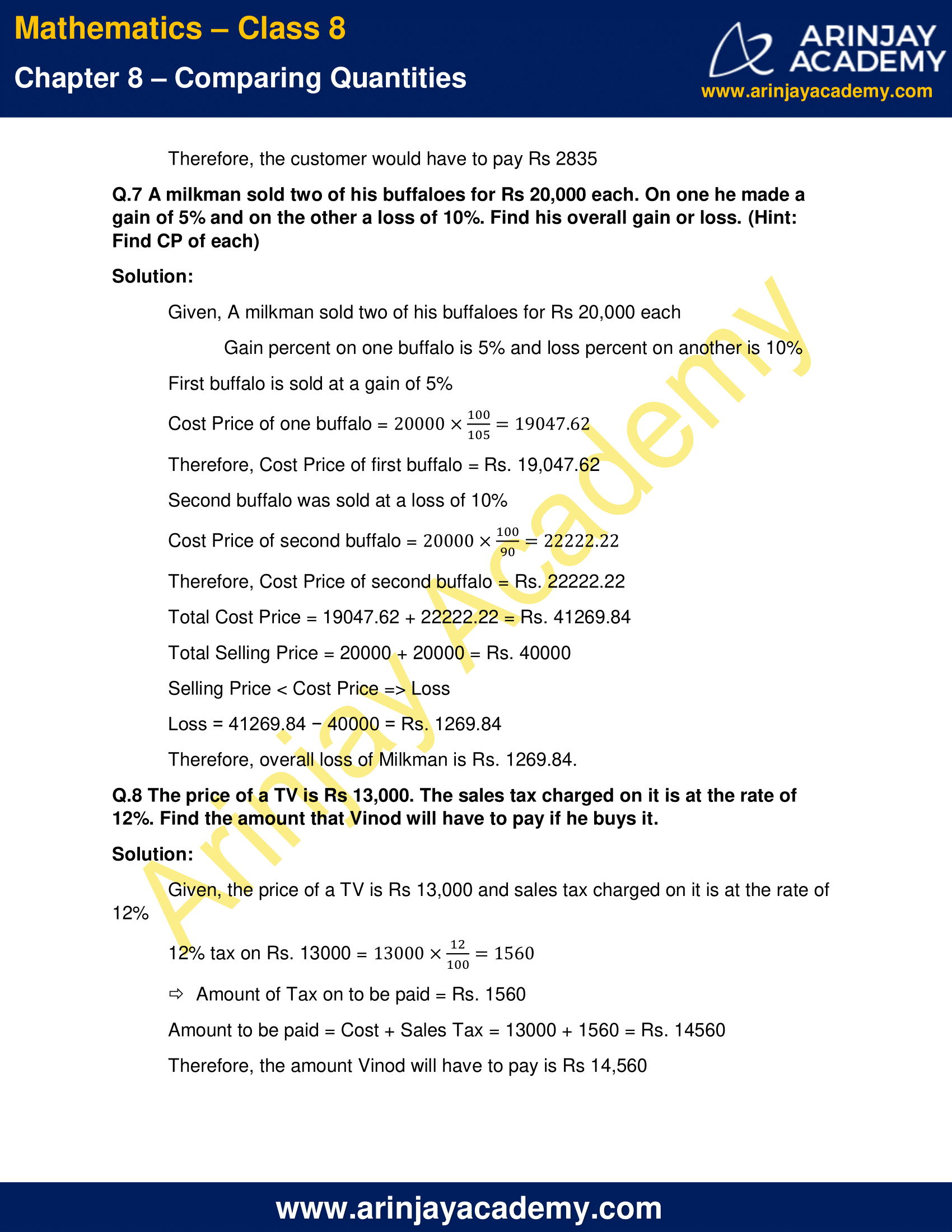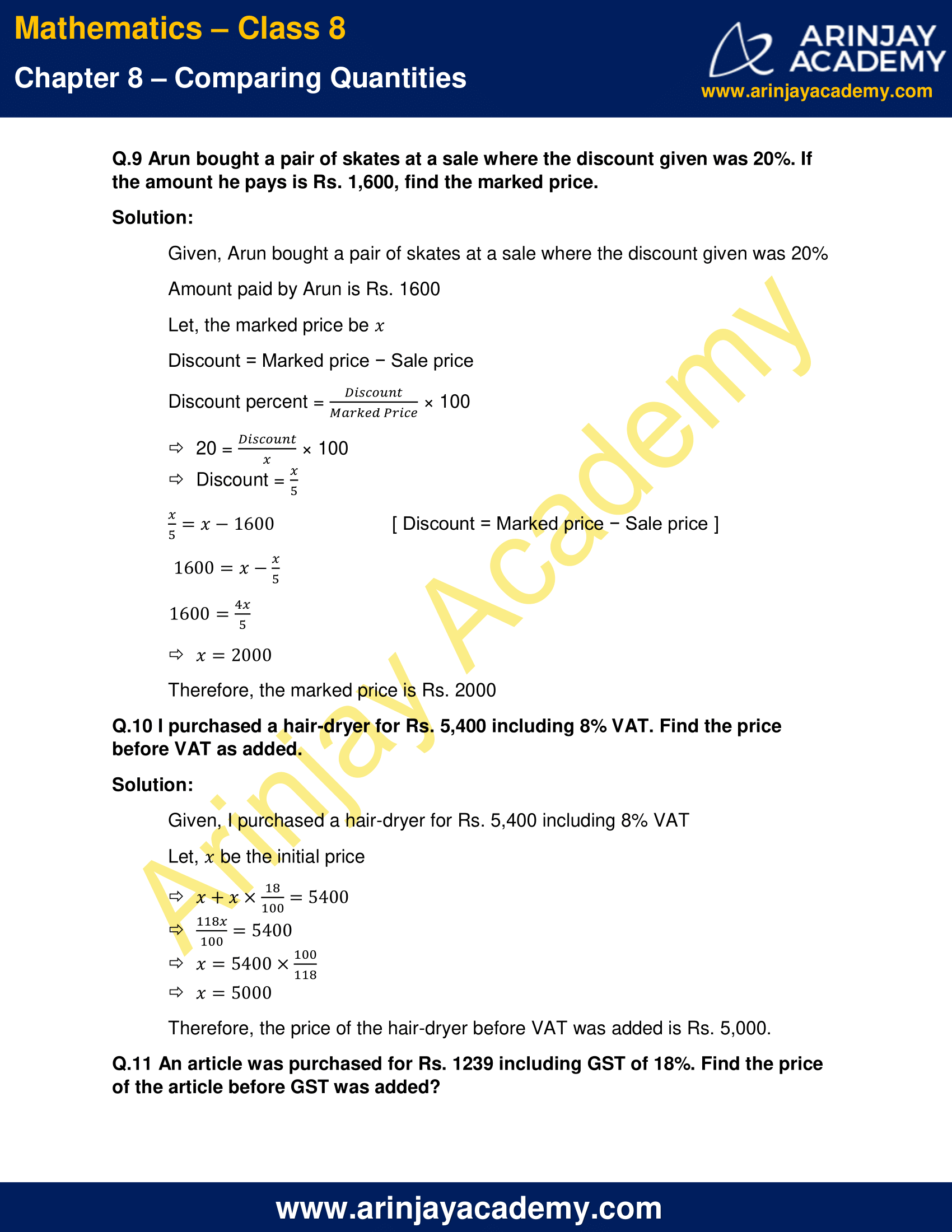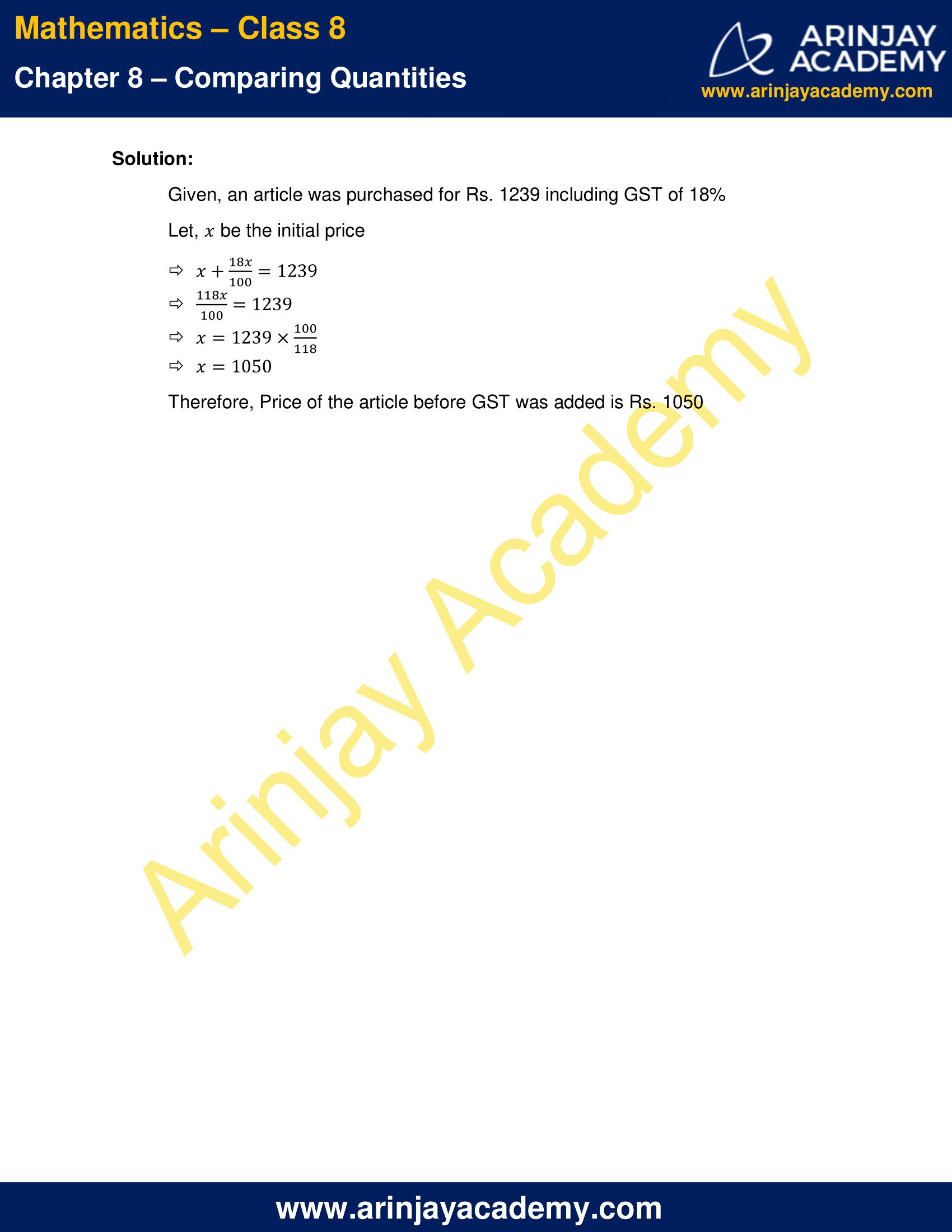NCERT Solutions for Class 8 Maths Chapter 8 Exercise 8.2 – Comparing Quantities

Q.1 A man got a 10% increase in his salary. If his new salary is Rs 1,54,000, find his original salary.

Solution:

Given, new salary is Rs 1,54,000.

Let, the original salary be ‘x’

10% increment in salary = (10/100) × x

New salary = Original salary + Increase in salary

154000 = x + (10/100) × x

x + (10/100) × x = 154000

x = 140000

Therefore, original salary is Rs. 1,40,000

Q.2 On Sunday 845 people went to the Zoo. On Monday only 169 people went. What is the percent decrease in the people visiting the Zoo on Monday?

Solution:

Given, on Sunday 845 people went to the zoo and on Monday only 169 people went.

Decrease in the number of people went to Zoo = 845 − 169 = 676

Percentage decrease = (676/845) x 100% = 80%

Therefore, percentage decrease in the people visiting the Zoo on Monday is 80%

Q.3 A shopkeeper buys 80 articles for Rs 2,400 and sells them for a profit of 16%. Find the selling price of one article.

Solution:

Given, A shopkeeper buys 80 articles for Rs 2,400 and sells them for a profit of 16%

Cost of one article = (2400/80) = Rs. 30

We know that profit percentage = (Profit/Cost Price) × 100

16 x (Profit/30) x 100

Profit = Rs. 4.80

Selling price = Cost price + Profit = 30 + 4.80 = Rs. 34.80

Therefore, the selling price of one article is Rs. 34.80

Q.4 The cost of an article was Rs 15,500. Rs 450 were spent on its repairs. If it is sold for a profit of 15%, find the selling price of the article.

Solution:

Given,

The cost of an article was Rs 15,500.

Rs 450 were spent on its repairs and sold for a profit of 15%

Total Cost Price of article = 15,500 + 450 = Rs. 15950

Profit percent = 15%

(Profit/Cost Price) x 100 = 15

(Profit/15950) x 100 = 15

Profit = (15950 × 15)/100

Profit = Rs. 2392.50

Therefore, Selling price of the article = Cost Price + Profit = 15950 + 2392.50

= Rs. 18342.50

Q.5 A VCR and TV were bought for Rs 8,000 each. The shopkeeper made a loss of 4% on  the VCR and a profit of 8% on the TV. Find the gain or loss percent on the whole transaction.

Solution:

Given, Cost Price of a VCR = Rs. 8000

The shopkeeper made a loss of 4% on VCR.

Loss = (Cost Price/100) × Loss percent

Loss = (8000/100) × 4

Loss = Rs. 320

Therefore, selling price of VCR is 8000 – 320 = Rs. 7680

Given, Cost Price of a TV = Rs 8000

The shopkeeper made a profit of 8 % on TV.

Profit = (Cost Price/100) × profit percent

Profit = (8000/100) × 8

Profit = Rs. 640

Therefore, Selling price of TV = 8000 + 640 = Rs. 8640

Total Cost Price = 8000 + 8000 = Rs. 16000

Total Selling Price = 7680 + 8640 = Rs. 16320

Selling Price > Cost Price =>  Profit

Therefore, Profit = 16320 − 16000 = Rs. 320

Profit Percentage = (16320 – 16000)/16000 x 100 = 2%

Therefore, the gain percent on whole transaction is 2%

Q.6 During a sale, a shop offered a discount of 10% on the marked prices of all the items. What would a customer have to pay for a pair of jeans marked at Rs 1450 and two shirts marked at Rs. 850 each?

Solution:

Given, during a sale, a shop offered a discount of 10% on marked prices of all items

Total marked price pair of jeans marked at Rs 1450 and two shirts marked at Rs. 850

each = 1450 + 2 × 850 = Rs. 3150

Discount percent = 10%

Discount = (10/100) x 3150 = Rs. 315

Selling Price = Cost Price – discount

Selling Price = 3150 – 315 = Rs. 2835

Therefore, the customer would have to pay Rs 2835

Q.7 A milkman sold two of his buffaloes for Rs 20,000 each. On one he made a gain of 5% and on the other a loss of 10%. Find his overall gain or loss. (Hint: Find CP of each)

Solution:

Given, A milkman sold two of his buffaloes for Rs 20,000 each

Gain percent on one buffalo is 5% and loss percent on another is 10%

First buffalo is sold at a gain of 5%

Cost Price of one buffalo = 20000 x (100/105) = 19047.62

Therefore, Cost Price of first buffalo = Rs. 19,047.62

Second buffalo was sold at a loss of 10%

Cost Price of second buffalo = 20000 x (100/90) = 22222.22

Therefore, Cost Price of second buffalo = Rs. 22222.22

Total Cost Price = 19047.62 + 22222.22 = Rs. 41269.84

Total Selling Price = 20000 + 20000 = Rs. 40000

Selling Price < Cost Price => Loss

Loss = 41269.84 − 40000 = Rs. 1269.84

Therefore, overall loss of Milkman is Rs. 1269.84.

Q.8 The price of a TV is Rs 13,000. The sales tax charged on it is at the rate of 12%. Find the amount that Vinod will have to pay if he buys it.

Solution:

Given, the price of a TV is Rs 13,000 and sales tax charged on it is at the rate of 12%

12% tax on Rs. 13000 = 13000 x (12/100) = 1560

Amount of Tax on to be paid = Rs. 1560

Amount to be paid = Cost + Sales Tax = 13000 + 1560 = Rs. 14560

Therefore, the amount Vinod will have to pay is Rs 14,560

Q.9 Arun bought a pair of skates at a sale where the discount given was 20%. If the amount he pays is Rs. 1,600, find the marked price.

Solution:

Given, Arun bought a pair of skates at a sale where the discount given was 20%

Amount paid by Arun is Rs. 1600

Let, the marked price be ‘x’

Discount = Marked price − Sale price

Discount percent = (Discount/Marked Price) × 100

20 = (Discount/x) × 100

Discount = (x/5)

(x/5) = x – 1600 [ Discount = Marked price − Sale price ]

1600 = x – (x/5)

1600 = (4x/5)

x = 2000

Therefore, the marked price is Rs. 2000

Q.10 I purchased a hair-dryer for Rs. 5,400 including 8% VAT. Find the price before VAT as added.

Solution:

Given, I purchased a hair-dryer for Rs. 5,400 including 8% VAT

Let, ‘x’ be the initial price

x + x × (18/100) = 5400

(118x/100) = 5400

x = 5400 x (100/118)

x = 5000

Therefore, the price of the hair-dryer before VAT was added is Rs. 5,000.

Q.11 An article was purchased for Rs. 1239 including GST of 18%. Find the price of the article before GST was added?

Solution:

Given, an article was purchased for Rs. 1239 including GST of 18%

Let, ‘x’ be the initial price

x + x × (18/100) =1239

(118x/100) = 1239

x = 1239 x (100/118)

x = 1050

Therefore, Price of the article before GST was added is Rs. 1050

The next Exercise for NCERT Solutions for Class 8 Maths Chapter 8 Exercise 8.3 – Comparing Quantities can be accessed by clicking here

Download NCERT Solutions for Class 8 Maths Chapter 8 Exercise 8.2 – Comparing Quantities